# Microstrip

Click here to go to our page on bends in transmission lines

Click here to go to our main page on microwave transmission lines

Click here to go to our page on calculating transmission line loss

Click here to go to our page on the history of microstrip

Microstrip is by far the most popular transmission line used in microwave engineering for circuit design.

## Types of microstrip media

The major advantage of microstrip over stripline is that all active components can be mounted on top of the board. The disadvantages are that when high isolation is required such as in a filter or switch, some external shielding may have to be considered. Given the chance, microstrip circuits can radiate, causing unintended circuit response. A minor issue with microstrip is that it is dispersive, meaning that signals of different frequencies travel at slightly different speeds. Microstrip supports a "quasi" TEM mode, because of its filling factor. One symptom of microstrip's non-TEM behavior is for coupled lines, the even and odd modes will not have the same phase velocity. This property is what causes the asymmetric frequency of microstrip bandpass filters, for example. For simple routing, you can pretend microstrip is TEM and get away with it.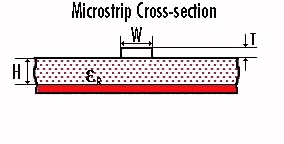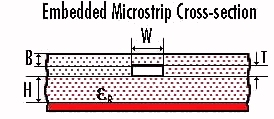Variants of microstrip include embedded microstrip and coated microstrip, both of which add some dielectric above the microstrip conductor. Anyone care to donate some material on these topics?

## Effective dielectric constant

Because part of the fields from the microstrip conductor exist in air, the effective dielectric constant "Keff" is somewhat less than the substrate's dielectric constant (also known as the relative permittivity). Thanks to Brian KC2PIT for reminding us the term "relative dielectric constant" is a tautology (not an oxymoron, thanks to Martin for clearing that up), only used by microwave morons!) According to Bahl and Trivedi, the effective dielectric constant εeff (a.k.a. Keff) of microstrip is calculated by: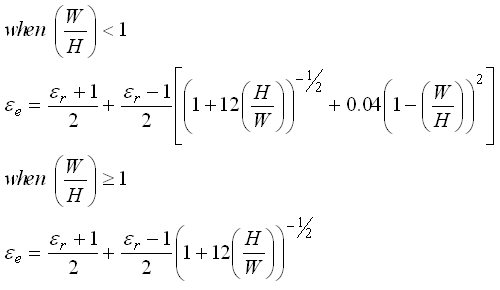All microstrip equations are approximate. The above equations ignore strip thickness, so we wouldn't recommend relying on them for critical designs on thick copper boards.

The effective dielectric constant is a seen to be a function of the ratio of the width to the height of a microstrip line (W/H), as well as the dielectric constant of the substrate material. Be careful, the way it is expressed here it is also a function of H/W!

We have a table of "hard" substrate material properties here, and "soft" substrate material properties here, in case you want to look up the dielectric constant of a specific material.

Note that there are separate solutions for cases where W/H is less than 1, and when W/H is greater than or equal to 1. These equations provide a reasonable approximation for εeff (effective dielectric constant). This calculation ignores strip thickness and frequency dispersion, but their effects are usually small.

Go to our microwave calculator page, our microstrip calculator does this calculation for you!

Here's calculator that was suggested to us that takes into account the metal thickness effect:

(link fixed May 1, 2008 thanks to Brian...)

https://emclab.mst.edu/resources/tools/pcb-trace-impedance-calculator/microstrip/

Let us know if you find it accurate (or not!)

### Wavelength

Wavelength for any transmission line can be calculated by dividing free space wavelength by the square root of the effective dielectric constant, which is explained above.

### Characteristic impedance

Characteristic impedance Z0 of microstrip is also a function of the ratio of the height to the width W/H (and ratio of width to height H/W) of the transmission line, and also has separate solutions depending on the value of W/H. According to Bahl and Trivedi, the characteristic impedance Z0 of microstrip is calculated by: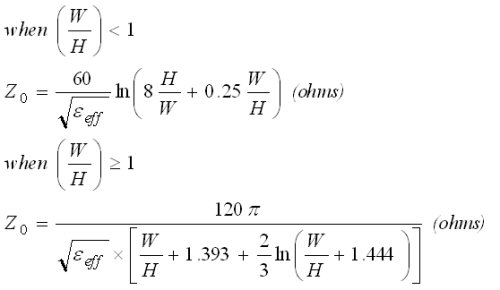Again, these equations are approximate, and don't take into account strip thickness. When strip thickness is a substantial fraction of substrate height, you should use a more accurate calculation. Suggestion: use Agilent ADS's Linecalc. One of these days we'll post some more accurate equations.

Go to our microwave calculator page, our microstrip calculator does this calculation for you!

It's time for a Microwaves101 Rule of Thumb!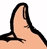For pure alumina (εR=9.8), the ratio of W/H for fifty-ohm microstrip is about 95%. That means on ten mil (254 micron) alumina, the width for fifty ohm microstrip will be about 9.5 mils (241 microns). On GaAs (εR=12.9), the W/H ratio for fifty ohms is about 75%. Therefore on four mil (100 micron) GaAs, fifty ohm microstrip will have a width of about 3 mils (75 microns). On PTFE-based soft board materials εR=2.2), W/H to get fifty ohms is about 3. Remember these!

#### Effect of metal thickness on calculations

Having a finite thickness of metal for conductor strips tends to increase the capacitance of the lines, which effects the εeff and Z0 calculations. We'll add this correction factor at a later date.

#### Effect of cover height on calculations

Having a lid in close proximity raises the capacitance per length, and therefore lowers the impedance. We suggest that if your impedance calculation is important, to use EDA software to make the final calculation on line widths!

## Cutoff frequencyBelow we present a microstrip rule of thumb, based on experience and not theory. In order to prevent higher-order transmission modes which might ruin your day, you should limit the thickness of your microstrip substrate to 10% of a wavelength. Examples of what this means: 15 mil alumina is good up to 25 GHz, 4 mil GaAs is good up to 82 GHz, and 5 mil quartz is good up to 121 GHz. There are formulas associated with calculating the exact cut-off frequencies, some day maybe we'll post them.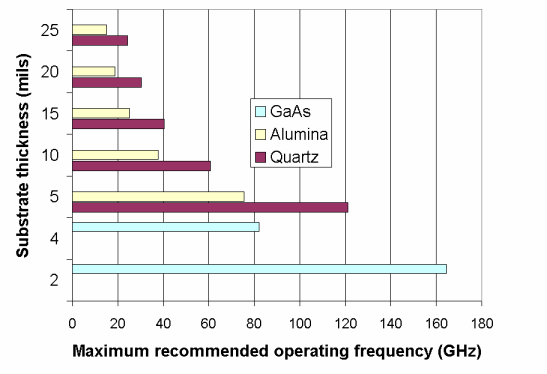Note to MMIC designers: increasing the height of a microstrip substrate decreases metal loss proportionately. It also increases the parasitic inductance associated with via holes. You may find that a two-mil substrate gives more gain than a four-mil substrate for the same active device, because of reduced inductance, and in many cases that "extra" gain will offset the loss associated with reduced strip widths. For switch designs, reduced substrate height means reduced switch isolation.

Note to GaN fab operators: as GaN-on-SiC moves into mainstream and up into millimeter-waves, it seems like most fabs are trying to duplicate the 50 and 100um (2 and 4 mil) thicknesses of GaAs substrates. If you consider that SiC has DK=10 versus GaAs DK of 12.9, you can get away with a 75um (three mil) process to serve all the way to 110 GHz. You will get better heat spreading and lower metal loss to boot.

## Microstrip loss calculations

This topic now has it's own page! Also, check out our page on transmission line loss calculations page.

## Dispersion

The effective dielectric constant (and therefore phase velocity and characteristic impedance) of microstrip is a slight function of frequency. This effect is not a big deal in most cases.

Here's our page on the topic of dispersion.

Our main discussion of microstrip dispersion is now here.

## References

 Reference: I. J. Bahl and D. K. Trivedi, "A Designer's Guide to Microstrip Line", Microwaves, May 1977, pp. 174-182. Go to our book section and buy a book on microstrip!

Author : Unknown Editor